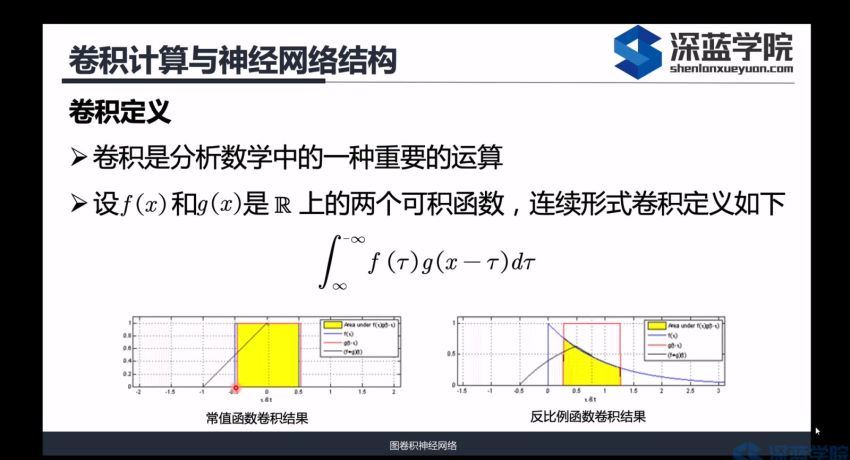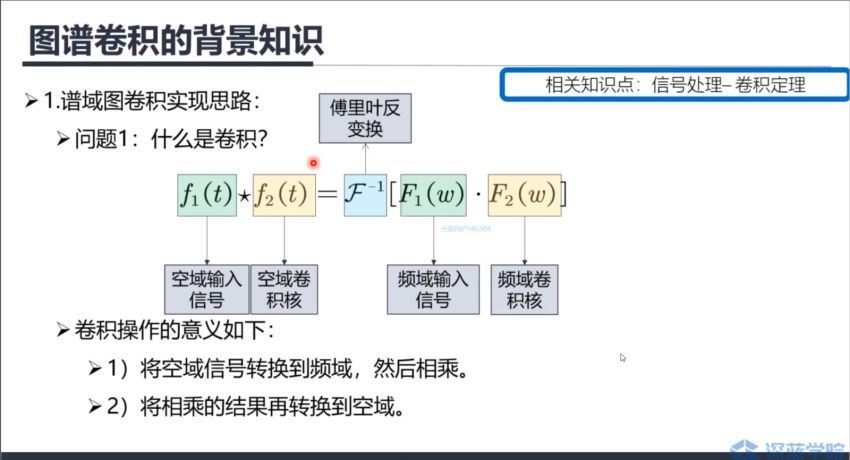# 深蓝图卷积神经网络，百度网盘分享## 课程文件目录：深蓝图卷积神经网络 体积大小：4.23G

chapter1卷积神经网络-从欧式空间到非欧式空间.mp4 [238.94M]

gcn第一节课.pdf [1.89M]

3.1-3.2 空域卷积.mp4 [356.77M]

3.1-3.2-3.3-3.4–l3空域图卷积介绍（一）.pdf [2.16M]

3.3-3.4 空域卷积.mp4 [132.89M]

3.5-3.6-v5.0过平滑现象.pdf [2.44M]

3.5图卷积网络回顾 空域图卷积2.mp4 [163.87M]

3.6过平滑现象.mp4 [310.24M]

【视频】环境搭建.mp4 [336.02M]

16：【视频】节点分类实践（上）.mp4 [229.28M]

16：【视频】节点分类实践（下）.mp4 [200.20M]

17：【视频】构造自己的数据集&查阅其他gcn方法.mp4 [309.06M]

19：第五章作业讲评.mp4 [61.49M]

code.rar [31.12M]

20：【视频】时序数据处理及建模.mp4 [349.37M]

21：【视频】基于pytorch的交通流量预测.mp4 [427.13M]

applications [201.82M]

combinatorial optimization [3.44M]

combinatorial optimization with graph convolutional networks and guided tree search(1).pdf [537.04K]

learning combinatorial optimization algorithms over graphs.pdf [2.91M]

graph generation [3.28M]

graph convolutional policy network for goal-directed molecular graph generation.pdf [517.97K]

molgan- an implicit generative model for small molecular graphs(1).pdf [1.10M]

netgan- generating graphs via random walks(1).pdf [1.67M]

image [40.43M]

image classification [1.69M]

few-shot learning with graph neural networks.pdf [1.69M]

interaction detection [1.10M]

structural-rnn- deep learning on spatio-temporal graphs.pdf [1.10M]

object detection [2.57M]

learning region features for object detection.pdf [1.68M]

relation networks for object detection.pdf [906.66K]

region classification [3.91M]

iterative visual reasoning beyond convolutions..pdf [3.91M]

semantic segmentation [24.98M]

3d graph neural networks for rgbd semantic segmentation.pdf [2.23M]

dynamic graph cnn for learning on point clouds.pdf [5.07M]

large-scale point cloud semantic segmentation with superpoint graphs.pdf [4.83M]

modeling polypharmacy side effects with graph convolutional networks.pdf [4.18M]

pointnet- deep learning on point sets for 3d classification and segmentation.pdf [8.66M]

social relationship understanding [0.00K]

graph-structured representations for visual question answering.pdf [3.74M]

out of the box- reasoning with graph convolution nets for factual visual question answering(1).pdf [2.45M]

knowledge graph [17.35M]

cross-lingual knowledge graph alignment via graph convolutional networks.pdf [432.63K]

deep reasoning with knowledge graph for social relationship understanding.pdf [2.76M]

dynamic graph generation network- generating relational knowledge from diagrams.pdf [1.19M]

knowledge transfer for out-of-knowledge-base entities – a graph neural network approach.pdf [355.22K]

modeling semantics with gated graph neural networks for knowledge base question answering.pdf [437.80K]

multi-label zero-shot learning with structured knowledge graphs.pdf [1.36M]

representation learning for visual-relational knowledge graphs.pdf [6.90M]

the more you know- using knowledge graphs for image classification.pdf [2.31M]

zero-shot recognition via semantic embeddings and knowledge graphs.pdf [1.63M]

science [130.64M]

a compositional object-based approach to learning physical dynamics.pdf [4.26M]

a note on learning algorithms for quadratic assignment with graph neural networks.pdf [340.40K]

a simple neural network module for relational reasoning.pdf [1.37M]

action schema networks- generalised policies with deep learning.pdf [1.67M]

adversarial attack on graph structured data.pdf [593.12K]

attend, infer, repeat- fast scene understanding with generative models.pdf [1.30M]

attention, learn to solve routing problems!.pdf [1.48M]

beyond categories- the visual memex model for reasoning about object relationships.pdf [618.71K]

combining neural networks with personalized pagerank for classification on graphs.pdf [483.25K]

constrained generation of semantically valid graphs via regularizing variational autoencoders.pdf [567.14K]

constructing narrative event evolutionary graph for script event prediction.pdf [654.87K]

conversation modeling on reddit using a graph-structured lstm.pdf [682.35K]

convolutional networks on graphs for learning molecular fingerprints.pdf [785.36K]

cross-sentence n-ary relation extraction with graph lstms.pdf [540.89K]

deep graph infomax.pdf [8.15M]

deepinf- modeling influence locality in large social networks.pdf [1.07M]

discovering objects and their relations from entangled scene representations.pdf [4.99M]

dynamic edge-conditioned filters in convolutional neural networks on graphs.pdf [567.07K]

effective approaches to attention-based neural machine translation.pdf [243.97K]

geometric matrix completion with recurrent multi-graph neural networks.pdf [6.99M]

graph convolutional matrix completion.pdf [732.99K]

graph convolutional neural networks for web-scale recommender systems.pdf [9.84M]

graph networks as learnable physics engines for inference and control.pdf [2.72M]

graphrnn- generating realistic graphs with deep auto-regressive models.pdf [2.43M]

hybrid approach of relation network and localized graph convolutional filtering for breast cancer subtype classification.pdf [2.52M]

hyperbolic attention networks.pdf [3.08M]

improved semantic representations from tree-structured long short-term memory networks.pdf [304.16K]

inference in probabilistic graphical models by graph neural networks.pdf [3.07M]

interaction networks for learning about objects, relations and physics.pdf [1.91M]

learning a sat solver from single-bit supervision.pdf [1.89M]

learning conditioned graph structures for interpretable visual question answering.pdf [8.48M]

learning deep generative models of graphs.pdf [2.31M]

learning graphical state transitions.pdf [1.47M]

learning human-object interactions by graph parsing neural networks.pdf [3.91M]

learning model-based planning from scratch.pdf [1.28M]

learning multiagent communication with backpropagation.pdf [4.13M]

learning to represent programs with graphs.pdf [421.90K]

metacontrol for adaptive imagination-based optimization.pdf [1.60M]

molecular graph convolutions- moving beyond fingerprints.pdf [2.08M]

nervenet learning structured policy with graph neural networks.pdf [3.11M]

neural combinatorial optimization with reinforcement learning.pdf [393.17K]

neural module networks.pdf [1.03M]

neural relational inference for interacting systems.pdf [2.83M]

protein interface prediction using graph convolutional networks.pdf [837.75K]

relational deep reinforcement learning.pdf [6.81M]

relational inductive bias for physical construction in humans and machines.pdf [1.00M]

relational neural expectation maximization- unsupervised discovery of objects and their interactions.pdf [1.15M]

self-attention with relative position representations.pdf [229.86K]

semi-supervised user geolocation via graph convolutional networks.pdf [1.13M]

situation recognition with graph neural networks.pdf [5.27M]

spatial temporal graph convolutional networks for skeleton-based action recognition.pdf [1.50M]

spatio-temporal graph convolutional networks- a deep learning framework for traffic forecasting.pdf [895.05K]

structured dialogue policy with graph neural networks.pdf [779.24K]

symbolic graph reasoning meets convolutions.pdf [3.23M]

traffic graph convolutional recurrent neural network- a deep learning framework for network-scale traffic learning and forecasting.pdf [1.45M]

translating embeddings for modeling multi-relational data.pdf [414.17K]

understanding kin relationships in a photo.pdf [1.44M]

vain- attentional multi-agent predictive modeling.pdf [423.97K]

visual interaction networks- learning a physics simulator from vide.o.pdf [5.41M]

text [6.70M]

sequence labeling [0.00K]

text classification [0.00K]

a graph-to-sequence model for amr-to-text generation.pdf [290.15K]

encoding sentences with graph convolutional networks for semantic role labeling.pdf [621.87K]

end-to-end relation extraction using lstms on sequences and tree structures.pdf [363.06K]

exploiting semantics in neural machine translation with graph convolutional networks.pdf [604.59K]

exploring graph-structured passage representation for multi-hop reading comprehension with graph neural networks..pdf [453.50K]

graph convolution over pruned dependency trees improves relation extraction.pdf [784.41K]

graph convolutional encoders for syntax-aware neural machine translation.pdf [346.90K]

graph convolutional networks for text classification.pdf [1.83M]

graph convolutional networks with argument-aware pooling for event detection.pdf [324.70K]

jointly multiple events extraction via attention-based graph.pdf [430.38K]

n-ary relation extraction using graph state lstm.pdf [455.67K]

recurrent relational networks.pdf [307.00K]

models [81.01M]

graph_type [16.10M]

directed graph [4.21M]

rethinking knowledge graph propagation for zero-shot learning.pdf [4.21M]

edge-informative graph [4.38M]

graph-to-sequence learning using gated graph neural networks.pdf [4.06M]

modeling relational data with graph convolutional networks.pdf [323.62K]

heterogeneous graphs [0.00K]

adaptive graph convolutional neural networks.pdf [803.92K]

graph capsule convolutional neural networks.pdf [1.93M]

graph neural networks for object localization.pdf [221.83K]

graph neural networks for ranking web pages.pdf [1.01M]

graph partition neural networks for semi-supervised classification.pdf [713.90K]

how powerful are graph neural networks-.pdf [678.30K]

mean-field theory of graph neural networks in graph partitioning.pdf [369.44K]

spectral networks and locally connected networks on graphs.pdf [1.86M]

others [30.67M]

a comparison between recursive neural networks and graph neural networks.pdf [247.20K]

a new model for learning in graph domains.pdf [177.61K]

celebritynet- a social network constructed from large-scale online celebrity images.pdf [16.33M]

contextual graph markov model- a deep and generative approach to graph processing.pdf [570.59K]

deep sets.pdf [5.11M]

deriving neural architectures from sequence and graph kernels.pdf [687.05K]

diffusion-convolutional neural networks.pdf [366.35K]

geometric deep learning on graphs and manifolds using mixture model cnns.pdf [7.23M]

propagation_type [20.75M]

attention [6.05M]

attention is all you need.pdf [2.10M]

graph attention networks.pdf [1.48M]

graph classification using structural attention.pdf [2.47M]

convolution [9.55M]

bayesian semi-supervised learning with graph gaussian processes.pdf [689.89K]

convolutional neural networks on graphs with fast localized spectral filtering.pdf [459.44K]

deep convolutional networks on graph-structured data.pdf [4.57M]

learning convolutional neural networks for graphs.pdf [639.85K]

spectral networks and deep locally connected.pdf [1.86M]

structure-aware convolutional neural networks.pdf [1.36M]

gate [1.16M]

gated graph sequence neural networks.pdf [748.16K]

sentence-state lstm for text representation.pdf [442.27K]

skip [3.99M]

representation learning on graphs with jumping knowledge networks.pdf [3.15M]

semi-supervised classification with graph convolutional networks.pdf [853.42K]

training methods [13.49M]

boosting [1.96M]

deeper insights into graph convolutional networks for semi-supervised learning.pdf [1.96M]

neighborhood sampling [1.95M]

adaptive sampling towards fast graph representation learning.pdf [579.95K]

fastgcn- fast learning with graph convolutional networks via importance sampling.pdf [358.35K]

inductive representation learning on large graphs.pdf [1.04M]

receptive field control [1.25M]

stochastic training of graph convolutional networks with variance reduction.pdf [1.25M]

covariant compositional networks for learning graphs.pdf [482.53K]

graphical-based learning environments for pattern recognition.pdf [335.92K]

hierarchical graph representation learning with differentiable pooling.pdf [2.31M]

knowledge-guided recurrent neural network learning for task-oriented action prediction.pdf [0.98M]

learning steady-states of iterative algorithms over graphs.pdf [3.09M]

neural networks for relational learning- an experimental comparison.pdf [1.15M]

survey [24.96M]

graph neural networks：a review of methods and applications.pdf [2.67M]

non-local neural networks.pdf [1.24M]

relational inductive biases, deep learning, and graph networks.pdf [8.99M]

the graph neural network model.pdf [1.43M]

a comprehensive survey on graph neural networks.pdf [1.80M]

computational capabilities of graph neural networks(1).pdf [1.28M]

deep learning on graphs- a survey.pdf [1.80M]

geometric deep learning- going beyond euclidean data.pdf [5.26M]

neural message passing for quantum chemistry.pdf [511.15K]

## 下载地址：

VIP会员免学豆下载，下载前请阅读文件目录，下载链接为百度云网盘，如网盘分享链接失效，可在下方评论，24小时内处理。

VIP优惠：免费